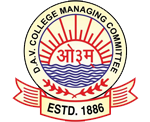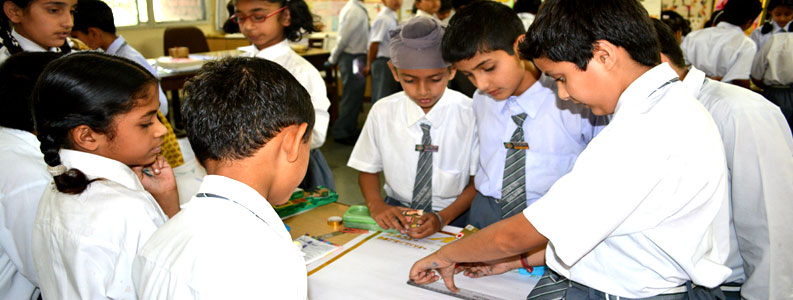Barmana (HP)
Latest News

Maths LabMathematics Laboratory is a place where students can learn and explore mathematical concepts and verify mathematical facts and theorems
through a variety of activities using different materials. These activities are carried out by the teacher or the students to explore, to learn, to stimulate interest and develop favorable attitude towards mathematics.DAV Sr Sec Public School Barmana is equipped with well furnished mathematics laboratory. The laboratory is designed to accommodatearound 45 to 50 students at a time where children can perform activities in groups. The lab is equipped with various types of models, charts on the life of great mathematician to inspire the students, mathematical kits to perform experiments etc. The walls of the lab is decorated by pasting interesting facts of maths puzzles, formulae charts etc.

It provides an opportunity to students to understand and internalize the basic mathematical concepts through concrete objects and situations.